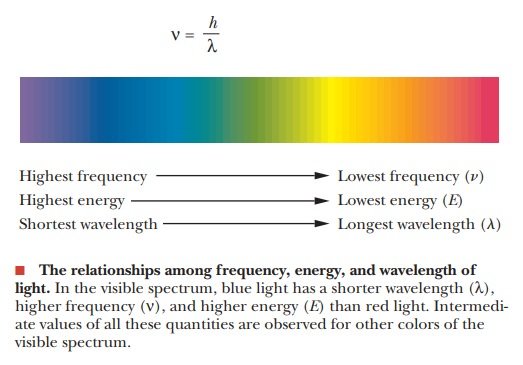Home | | Biochemistry | The Relationship between Wavelength and Energy of Light

# The Relationship between Wavelength and Energy of Light

A well-known equation relates the wavelength and energy of light, a point of crucial importance for our purposes.

The Relationship between Wavelength and Energy of Light

A well-known equation relates the wavelength and energy of light, a point of crucial importance for our purposes. Max Planck established in the early 20th century that the energy of light is directly proportional to its frequency.

E = hν

whereE is energy, h is a constant (PlanckÕs constant), and ν is the frequency of the light. The wavelength of light is related to the frequency.whereλ is wavelength, ν is frequency, and c is the velocity of light. We can rewrite the expression for the energy of light in terms of wavelength rather than frequency.Light of shorter wavelength (higher frequency) is higher in energy than light of longer wavelength (lower frequency).

Study Material, Lecturing Notes, Assignment, Reference, Wiki description explanation, brief detail
Biochemistry: Photosynthesis : The Relationship between Wavelength and Energy of Light |